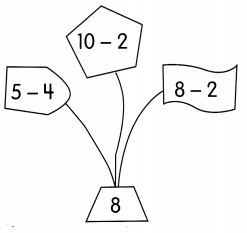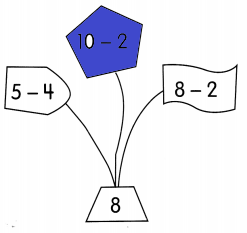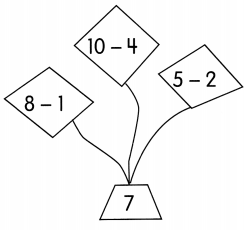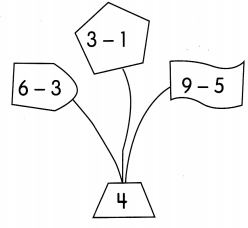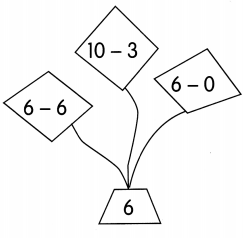# Math in Focus Grade 1 Chapter 4 Practice 1 Answer Key Ways to Subtract

This handy Math in Focus Grade 1 Workbook Answer Key Chapter 4 Practice 1 Ways to Subtract detailed solutions for the textbook questions.

## Math in Focus Grade 1 Chapter 4 Practice 1 Answer Key Ways to Subtract

Cross out to subtract. Then circle the answer.

Example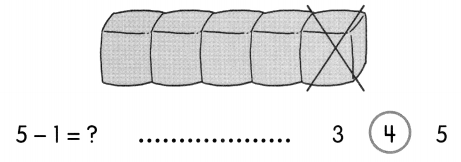Question 1.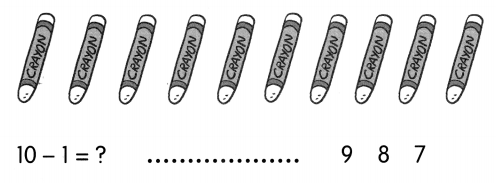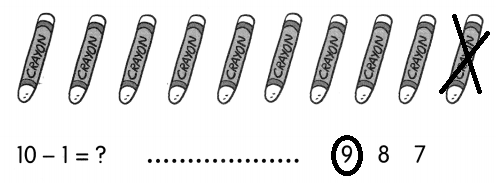Explanation:
The difference between 10 and 1 is 9

Question 2.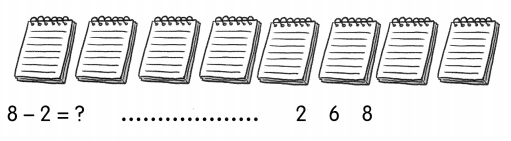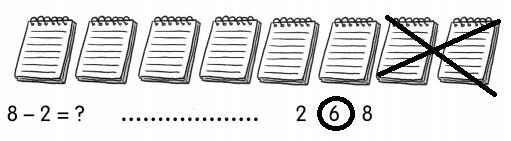Explanation:
The difference between 8 and 2 is 6

Write a subtraction sentence for each picture.

Example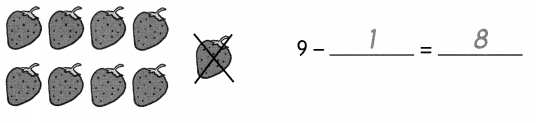Question 3.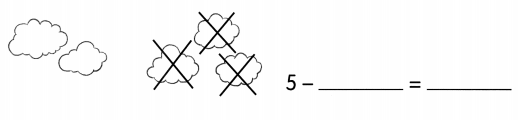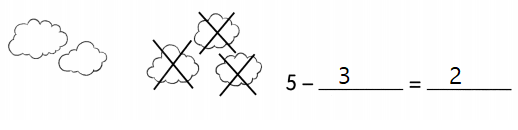Explanation:
The difference between 5 and 3 is 2

Question 4.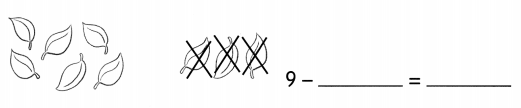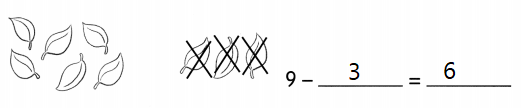Explanation:
The difference between 9 and 3 is 6

Question 5.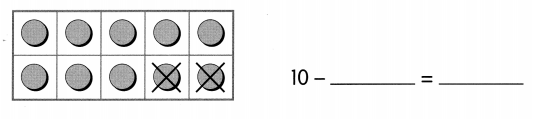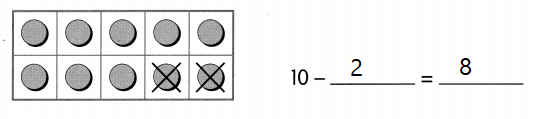Explanation:
The difference between 10 and 2 is 8

Question 6.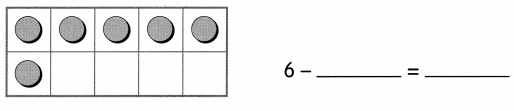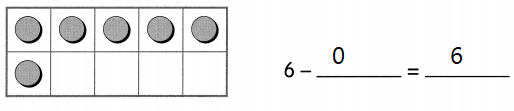Explanation:
The difference between 6 and 0 is 6

Complete.

Example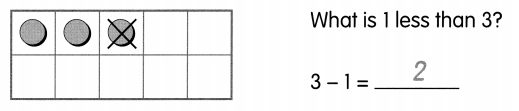Question 7.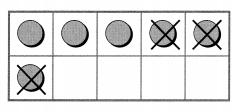What is 3 less than 6?
6 – 3 = ____
6 – 3 = 3
Explanation:
The difference between 6 and 3 is 3

Cross out to subtract.
Then write the subtraction sentence.

Example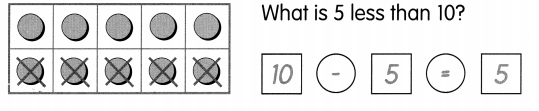Question 8.
What is 4 less than 7?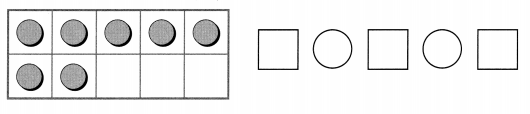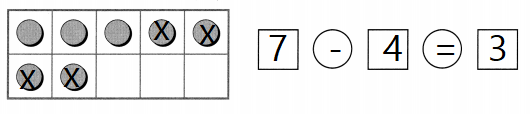Explanation:
The difference between 7 and 4 is 3

Cross out to subtract.
Then write the subtraction sentence.

Question 9.
What is 2 less than 9?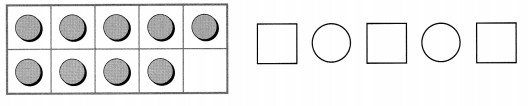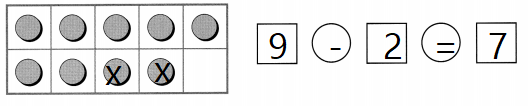Explanation:
The difference between 9 and 2  is 7

Subtract. Count on from the number that is less. Fill in the blanks.

Example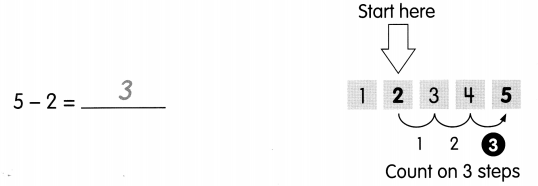Question 10.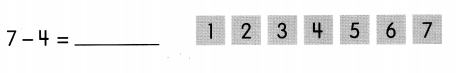Question 11.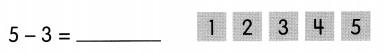Question 12.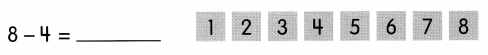Count back from the greater number to subtract. Fill in the blanks.

Example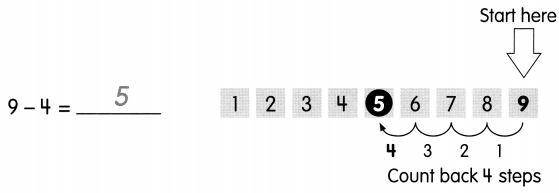Question 13.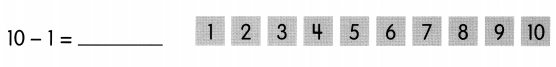Question 14.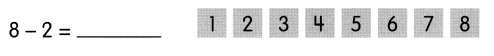Question 15.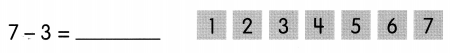Question 16.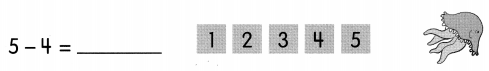Question 17.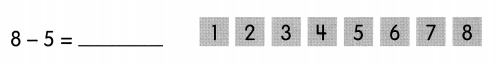Question 18.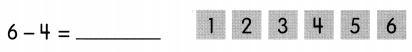Color the correct shape.

Example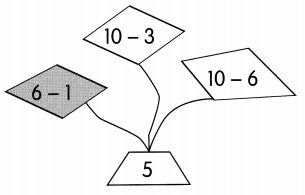Question 19.